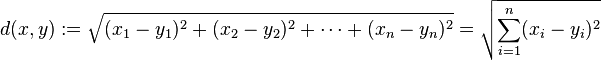# Python机器学习k-近邻算法(K Nearest Neighbor)实例详解

8 6月 , 2019

### 工作原理• 如果k=3，那么离绿色点最近的有2个红色三角形和1个蓝色的正方形，这3个点投票，于是绿色的这个待分类点属于红色的三角形。
• 如果k=5，那么离绿色点最近的有2个红色三角形和3个蓝色的正方形，这5个点投票，于是绿色的这个待分类点属于蓝色的正方形。

### kNN算法实施

1. 计算已知类型类别数据集中的点与当前点之间的距离
2. 按照距离递增次序排序
3. 选取与当前点距离最小的k个点
4. 确定前k个点所在类别的出现频率
5. 返回前k个点出现频率最高的类别作为当前点的预测分类python代码实现

``````# -*- coding:utf-8 -*-
#! python2
import numpy as np
import operator
# 训练集
data_set = np.array([[1., 1.1],
[1.0, 1.0],
[0., 0.],
[0, 0.1]])
labels = ['A', 'A', 'B', 'B']
def classify_knn(in_vector, training_data, training_label, k):
"""
:param in_vector: 待分类向量
:param training_data: 训练集向量
:param training_label: 训练集标签
:param k: 选择最近邻居的数目
:return: 分类器对 in_vector 分类的类别
"""
data_size = training_data.shape # .shape 返回二维数组的行数
diff_mat = np.tile(in_vector, (data_size, 1)) - data_set # np.tile(array, (3, 2)) 对 array 进行 3×2 扩展为二维数组
sq_diff_mat = diff_mat ** 2
sq_distances = sq_diff_mat.sum(axis=1) # .sum(axis=1) 矩阵以列求和
# distances = sq_distances ** 0.5 # 主要是通过比较求最近点,所以没有必要求平方根
distances_sorted_index = sq_distances.argsort() # .argsort() 对array进行排序 返回排序后对应的索引
class_count_dict = {} # 用于统计类别的个数
for i in range(k):
label = training_label[distances_sorted_index[i]]
try:
class_count_dict[label] += 1
except KeyError:
class_count_dict[label] = 1
class_count_dict = sorted(class_count_dict.iteritems(), key=operator.itemgetter(1), reverse=True) # 根据字典的value值对字典进行逆序排序
return class_count_dict
if __name__ == '__main__':
vector = [0, 0] # 待分类数据集
print classify_knn(in_vector=vector, training_data=data_set, training_label=labels, k=3)
``````

### 算法评价

• 优点：精度高、对异常值不敏感、无数据输入假定
• 缺点：计算复杂度高、空间复杂度高
• 使用数据范围：数据型和标称型
• 适用：kNN方法通常用于一个更复杂分类算法的一部分。例如，我们可以用它的估计值做为一个对象的特征。有时候，一个简单的kNN算法在良好选择的特征上会有很出色的表现。

[附源代码]

#### 您可能感兴趣的文章:

KNN算法介绍

KNN是一种监督学习算法，通过计算新数据与训练数据特征值之间的距离，然后选取K(K>=1)个距离最近的邻居进行分类判(投票法)或者回归。若K=1，新数据被简单分配给其近邻的类。

KNN算法实现过程

(1)选择一种距离计算方式,

(2)按照距离递增次序进行排序，选取与当前距离最小的k个点；

(3)对于离散分类，返回k个点出现频率最多的类别作预测分类；对于回归则返回k个点的加权值作为预测值；

(1)数据的所有特征都要做可比较的量化

(2)样本特征要做归一化处理

(3)需要一个距离函数以计算两个样本之间的距离

(4)确定K的值

K值选的太大易引起欠拟合，太小容易过拟合。交叉验证确定K值。

KNN分类

KNN回归

KNN回归是取K个邻居类标签值得加权作为新数据点的预测值。

(1)KNN算法的优点

• 1.简单、有效。
• 2.重新训练的代价较低(类别体系的变化和训练集的变化，在Web环境和电子商务应用中是很常见的)。
• 3.计算时间和空间线性于训练集的规模(在一些场合不算太大)。
• 4.由于KNN方法主要靠周围有限的邻近的样本，而不是靠判别类域的方法来确定所属类别的，因此对于类域的交叉或重叠较多的待分样本集来说，KNN方法较其他方法更为适合。
• 5.该算法比较适用于样本容量比较大的类域的自动分类，而那些样本容量较小的类域采用这种算法比较容易产生误分。

(2)KNN算法缺点

• 1.KNN算法是懒散学习方法(lazy
learning,基本上不学习)，一些积极学习的算法要快很多。
• 2.类别评分不是规格化的(不像概率评分)(???)。
• 3.输出的可解释性不强，例如决策树的可解释性较强。
• 4.该算法在分类时有个主要的不足是，当样本不平衡时，如一个类的样本容量很大，而其他类样本容量很小时，有可能导致当输入一个新样本时，该样本的K个邻居中大容量类的样本占多数。该算法只计算最近的邻居样本，某一类的样本数量很大，那么或者这类样本并不接近目标样本，或者这类样本很靠近目标样本。无论怎样，数量并不能影响运行结果。可以采用权值的方法(和该样本距离小的邻居权值大)来改进。
• 5.计算量较大。目前常用的解决方法是事先对已知样本点进行剪辑，事先去除对分类作用不大的样本。

KNN实现

``````import numpy as np
import operator
import matplotlib
import matplotlib.pyplot as plt
from os import listdir
def Create_DataSet():
group = np.array([[1.0, 1.1],[1.0,1.0],[0,0],[0,0.1]])
labels = ['A','A','B','B']
return group,labels
``````